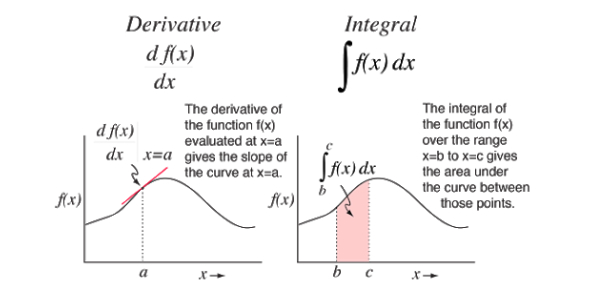# Test Your Knowledge With The Derivative Rules Assessment Test

10 Questions | Total Attempts: 291SettingsThink you are a Maths expert? How much do you know about Differentiation? To find many derivatives, we follow rules namely the multiplication by constant, Power Rule, Sum Rule, Difference Rule, Product Rule, Reciprocal Rule, and the three Chain Rules. Take this assessment test to find out more.

• 1.
To find the antiderivative for a function, you...
• A.

Reverse the process of differentiation

• B.

Derive the function

• C.

Multiply the function

• D.

Differentiate

• 2.
Which is one of a rule of differentiation?
• A.

Base rule

• B.

Sum rule

• C.

Light rule

• D.

Base law

• 3.
The action of computing a derivative is...
• A.

Calculation

• B.

Integration

• C.

Computation

• D.

Differentiation

• 4.
A measure of the rate at which the value of a function changes with respect to the change of the variable is?
• A.

Derived

• B.

Derivative of the function

• C.

Exponent

• D.

Base of function

• 5.
A horizontal line with a slope of zero has a derivative of...
• A.

0

• B.

1

• C.

2

• D.

1.1

• 6.
A differentiable function whose derivative is equal to the original function is the...
• A.

Function

• B.

Antiderivative

• C.

Base

• D.

Rule

• 7.
Which of the following has a defined limit?
• A.

Integral

• B.

Curve

• C.

Line

• D.

Slope

• 8.
What is the product-based counterpart of the usual sum-based integral of classical calculus?
• A.

Integration

• B.

Function

• C.

Product integral

• D.

Differentiation

• 9.
The fundamental theorem of calculus says that antiderivatives and indefinite integrals are...
• A.

The same

• B.

Different

• C.

Zero

• D.

Minus One

• 10.
The inverse operation of differentiation is...
• A.

Integral

• B.

Rule

• C.

Antiderivative

• D.

FunctionBack to top